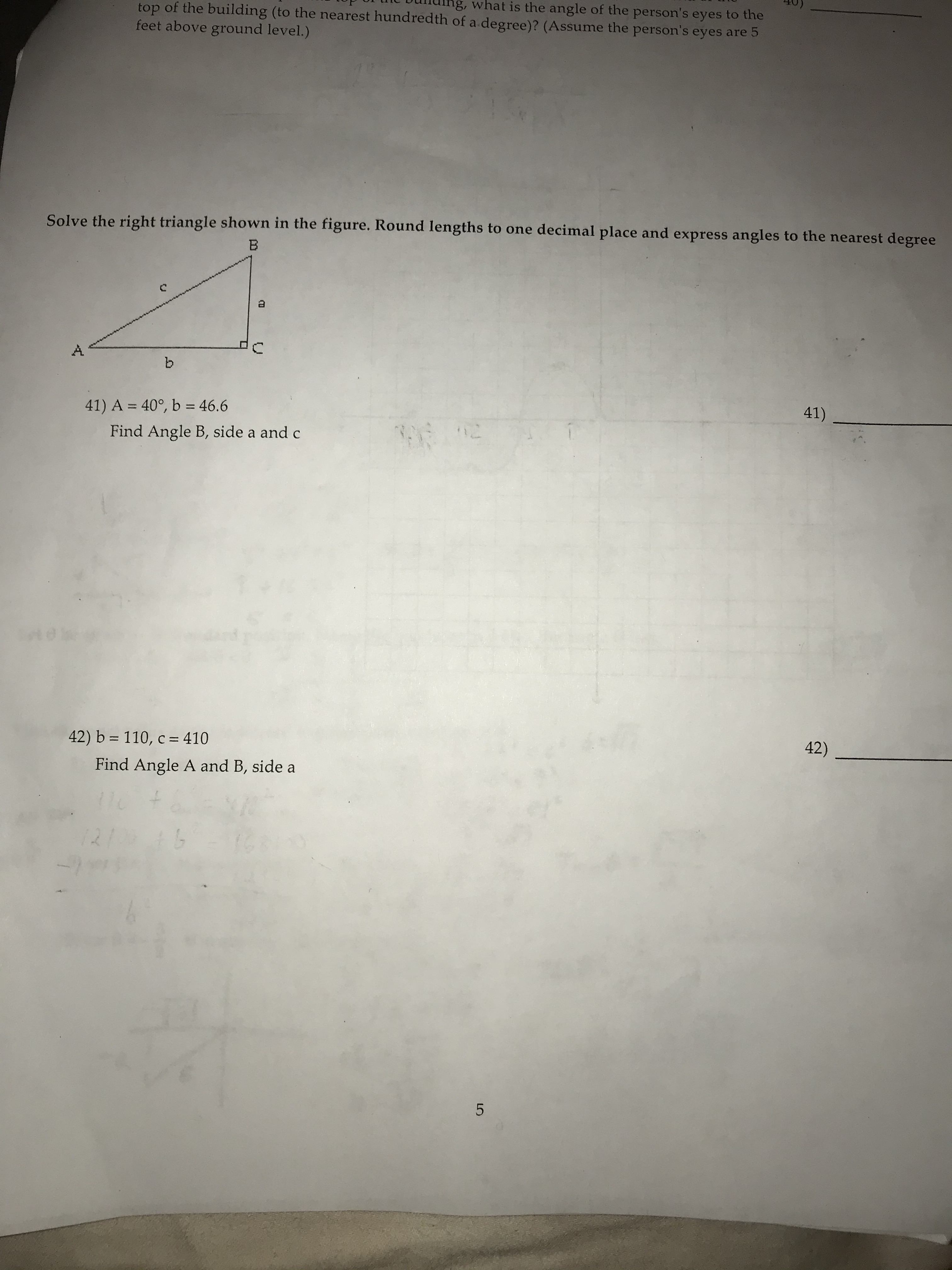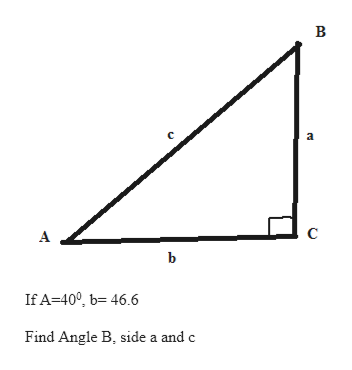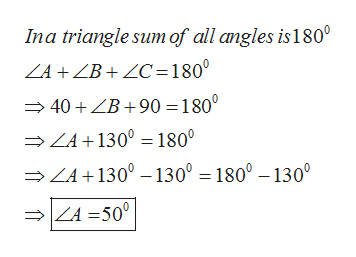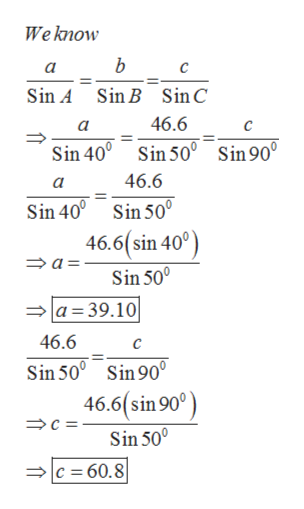# ng, what is the angle of the person's eyes to thetop of the building (to the nearest hundredth of a degree)? (Assume the person's eyes are 5feet above ground level.)Solve the right triangle shown in the figure. Round lengths to one decimal place and express angles to the nearest degreeb.41)41) A = 40°, b = 46.6%3D%3DFind Angle B, side a and c42)42) b = 110, c = 410Find Angle A and B, side a1211A.

Question
2 views

Solve the right traingle shown in the figure. Round lengths to one decimal place and express to the nearest degree

A = 40degrees , b = 46.6 Find Angle B, side a and chelp_outlineImage Transcriptioncloseng, what is the angle of the person's eyes to the top of the building (to the nearest hundredth of a degree)? (Assume the person's eyes are 5 feet above ground level.) Solve the right triangle shown in the figure. Round lengths to one decimal place and express angles to the nearest degree b. 41) 41) A = 40°, b = 46.6 %3D %3D Find Angle B, side a and c 42) 42) b = 110, c = 410 Find Angle A and B, side a 1211 A. fullscreen
check_circle

Step 1

Solve the right triangle ABChelp_outlineImage TranscriptioncloseB a A If A=40°, b= 46.6 Find Angle B, side a and c fullscreen
Step 2

To find angle Bhelp_outlineImage TranscriptioncloseIna triangle sum of all angles is180° ZA + ZB + ZC=180° = 40 +ZB +90 =180° → ZA+130° = 180° → ZA + 130° – 130° = 180° – 130° = ZA =50° fullscreen
Step 3

Side a and...help_outlineImage TranscriptioncloseWe know a Sin A Sin B SinC 46.6 a Sin 40° Sin 50° Sin90° 46.6 a Sin 50° Sin 40° 46.6(sin 40°) → a= Sin 50° |a=39.10 46.6 Sin 50° Sin 90° 46.6(sin 90°) Sin 50° = 60.8 fullscreen

### Want to see the full answer?

See Solution

#### Want to see this answer and more?

Solutions are written by subject experts who are available 24/7. Questions are typically answered within 1 hour.*

See Solution
*Response times may vary by subject and question.
Tagged in

### Trigonometric Ratios